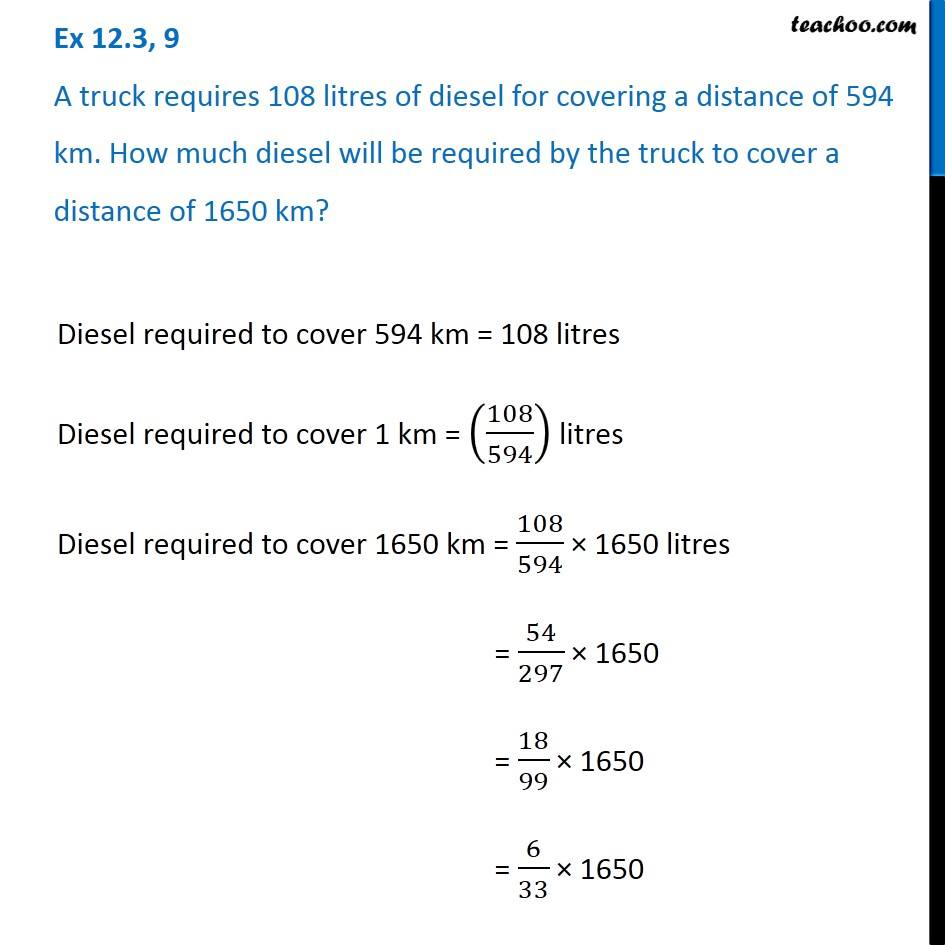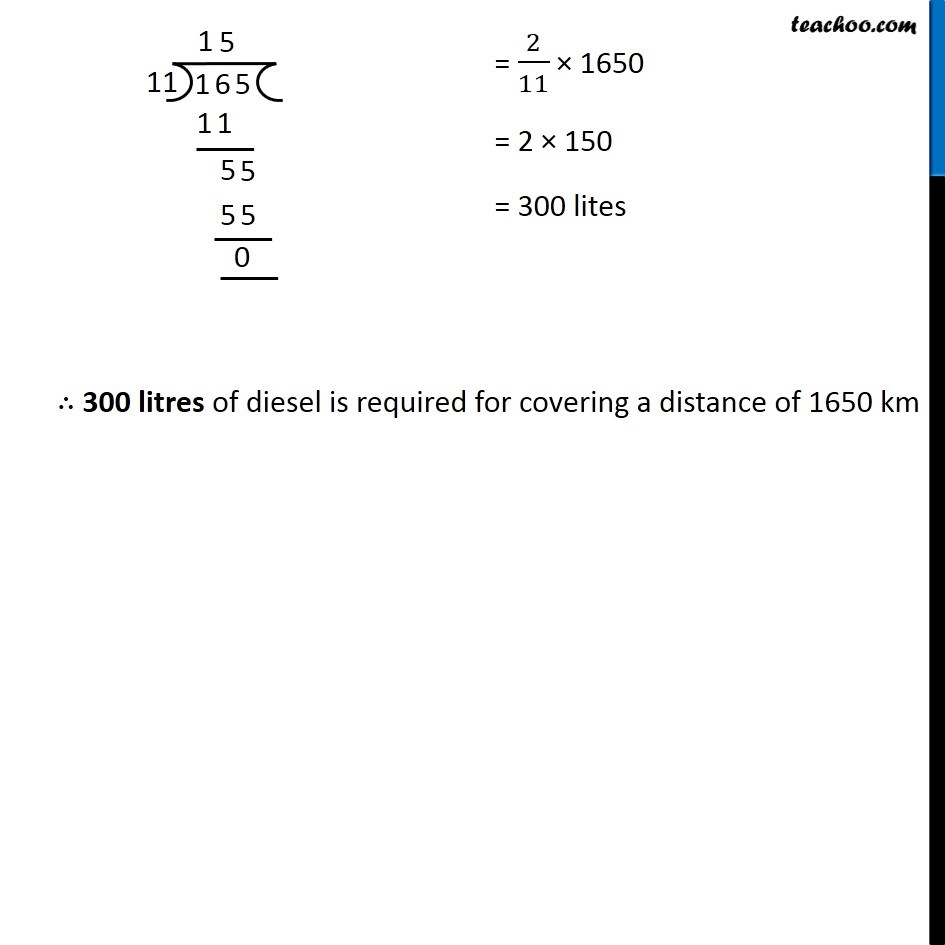1. Chapter 12 Class 6 Ratio And Proportion
2. Concept wise
3. Unitary Method

Transcript

Ex 12.3, 9 A truck requires 108 litres of diesel for covering a distance of 594 km. How much diesel will be required by the truck to cover a distance of 1650 km? Diesel required to cover 594 km = 108 litres Diesel required to cover 1 km = (108/594) litres Diesel required to cover 1650 km = 108/594 × 1650 litres = 54/297 × 1650 = 18/99 × 1650 = 6/33 × 1650 = 2/11 × 1650 = 2 × 150 = 300 lites ∴ 300 litres of diesel is required for covering a distance of 1650 km

Unitary Method